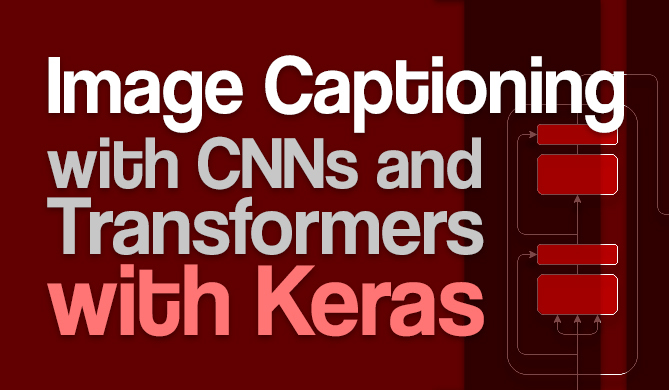Classify Images with Pre-Trained Model using Keras

# Classify Images with Pre-Trained Model using KerasComputer Vision models have come a long way - and you can leverage existing models, pre-trained on large corpora of data, and just plug them into your prediction pipeline.

While fine-tuning a network is the best way to go - importing an existing model and running predictions from the get-go is a valid use-case in early stages of prototyping or just for the sake of trying a model out. For a detailed in-depth guide on fine-tuning via Transfer Learning - read our free lesson on "Transfer Learning for Computer Vision with Keras"!

That being said - the main variable is how you load an image in, the most notable ways being using OpenCV, PIL and the Keras preprocessing helper methods.

### OpenCV

OpenCV is to Computer Vision, what Scikit-Learn is to Machine Learning. It's a popular, well-rounded library, widely used in the industry, with a slightly steeper learning curve than image processing libraries like PIL.

We'll create a proprietary helper function to obtain and convert an image via a URL into a NumPy array and then classify it using a pre-trained model from keras.applications:

from tensorflow import keras
# preprocess_input is a pass-through function for EffNets
from keras.applications.efficientnet import preprocess_input, decode_predictions

import urllib
import cv2
import numpy as np

def url_to_array(url):
req = urllib.request.urlopen(url)
arr = cv2.imdecode(arr, -1)
arr = cv2.cvtColor(arr, cv2.COLOR_BGR2RGB)
arr = cv2.resize(arr, (224, 224))
return arr

img = url_to_array(url)
img_batch = np.expand_dims(img, 0)

effnet = keras.applications.EfficientNetV2B0(weights='imagenet', include_top=True)
pred = effnet.predict(img_batch)
print(decode_predictions(pred))
# [[('n02133161', 'American_black_bear', 0.8694465), ('n02134418', 'sloth_bear', 0.03677345), ('n02132136', 'brown_bear', 0.020618025), ('n02134084', 'ice_bear', 0.0011013364), ('n02128925', 'jaguar', 0.00081196934)]]


Let's visualize the image with the prediction:

plt.imshow(img)
plt.title(f'Class: {decode_predictions(pred)}\nConfidence: {decode_predictions(pred)*100}%')
plt.show()


### Keras Preprocessing

Keras offers preprocessing helper functions and classes which allows us to load and prepare images as NumPy arrays:

from tensorflow import keras
# preprocess_input is a pass-through function for EffNets
from keras.applications.efficientnet import preprocess_input, decode_predictions
from tensorflow.keras.preprocessing import image

import urllib.request
import matplotlib.pyplot as plt
import numpy as np

# Public domain image
urllib.request.urlretrieve(url, 'bear.jpg')

# Load image and resize (doesn't keep aspect ratio)
# Turn to array of shape (224, 224, 3)
img = image.img_to_array(img)
# Expand array into (1, 224, 224, 3)
img_batch = np.expand_dims(img, 0)
# Preprocess for models that have specific preprocess_input() function
# img_preprocessed = preprocess_input(img)

# Load model and run prediction
effnet = keras.applications.EfficientNetV2B0(weights='imagenet', include_top=True)
pred = effnet.predict(img_batch)
print(decode_predictions(pred))
# [[('n02133161', 'American_black_bear', 0.87167954), ('n02134418', 'sloth_bear', 0.035227217), ('n02132136', 'brown_bear', 0.021057261), ('n02134084', 'ice_bear', 0.0011267527), ('n02128925', 'jaguar', 0.0007899802)]]


## Get free courses, guided projects, and more

Let's plot it:

plt.imshow(img.astype('int'))
plt.title(f'Class: {decode_predictions(pred)}\nConfidence: {decode_predictions(pred)*100}%')
plt.show()


### PIL

Finally, PIL is a generally popular image processing library and can naturally be used to load images as NumPy arrays:

from tensorflow import keras
# preprocess_input is a pass-through function for EffNets
from keras.applications.efficientnet import preprocess_input, decode_predictions
from tensorflow.keras.preprocessing import image

import PIL

import urllib.request
import matplotlib.pyplot as plt
import numpy as np

# Public domain image
img = PIL.Image.open(urllib.request.urlopen(url))
img = img.resize((224, 224))
img_batch = np.expand_dims(img, 0)

# Load model and run prediction
effnet = keras.applications.EfficientNetV2B0(weights='imagenet', include_top=True)
pred = effnet.predict(img_batch)
print(decode_predictions(pred))
# [[('n02133161', 'American_black_bear', 0.82402), ('n02134418', 'sloth_bear', 0.07482512), ('n02132136', 'brown_bear', 0.01921546), ('n02483708', 'siamang', 0.001174309), ('n02134084', 'ice_bear', 0.00081004837)]]


Let's plot it:

plt.imshow(img)
plt.title(f'Class: {decode_predictions(pred)}\nConfidence: {decode_predictions(pred)*100}%')
plt.show()

Last Updated: July 3rd, 2022
David LandupAuthor

Entrepreneur, Software and Machine Learning Engineer, with a deep fascination towards the application of Computation and Deep Learning in Life Sciences (Bioinformatics, Drug Discovery, Genomics), Neuroscience (Computational Neuroscience), robotics and BCIs.

Great passion for accessible education and promotion of reason, science, humanism, and progress.Course

### Practical Deep Learning for Computer Vision with Python

# tensorflow# machine learning# python# pytorch

When you look down at your coffee mug, you're not at all surprised by the object in front of you. You've been able to do...DetailsProject

### Image Captioning with CNNs and Transformers with Keras

# artificial intelligence# deep learning# python# nlp

In 1974, Ray Kurzweil's company developed the &quot;Kurzweil Reading Machine&quot; - an omni-font OCR machine used to read text out loud. This machine...David Landup
Details